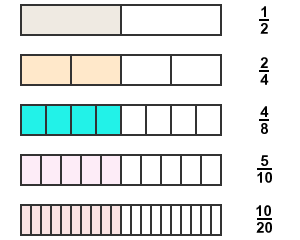# Equal fractions

The fractions which have same quantity are called equivalent fractions.

Two are more fractions often have same fractional quantity but the numerical representation of them are different. However, they are equal in the point of whole fractional quantity. Hence, they are also called as equal fractions.

## Example

Consider five rectangles and divide each rectangle into different number of equal parts.1. Divide the first rectangle into two equal parts. The fraction of selection portion is $\frac{1}{2}$.
2. Divide the second rectangle into four equal parts. The fraction of selection portion is $\frac{2}{4}$.
3. Divide the third rectangle into eight equal parts. The fraction of selection portion is $\frac{4}{8}$.
4. Divide the fourth rectangle into ten equal parts. The fraction of selection portion is $\frac{5}{10}$.
5. Divide the fifth rectangle into 20 equal parts and the fraction of selection portion is $\frac{10}{20}$.

$\frac{1}{2}$, $\frac{2}{4}$, $\frac{4}{8}$, $\frac{5}{10}$ and $\frac{10}{20}$ are five fractions. All these fractions contain different numerators and also different denominators in their representation.

It seem they are different numerically but they are not. Actually, the numerical value of all of them is same because the selective quantity in every whole quantity is same.

$\frac{1}{2}=\frac{2}{4}=\frac{4}{8}=\frac{5}{10}=\frac{10}{20}=0.5$

The value of each fraction is 0.5. it clears that even though the numerical representation of two or more fractions is different, they are called as equal fractions if they have same fractional quantity.

Latest Math Topics
Latest Math Problems
Email subscription
Math Doubts is a best place to learn mathematics and from basics to advanced scientific level for students, teachers and researchers. Know more
Follow us on Social Media
###### Math Problems

Learn how to solve easy to difficult mathematics problems of all topics in various methods with step by step process and also maths questions for practising.

Learn more